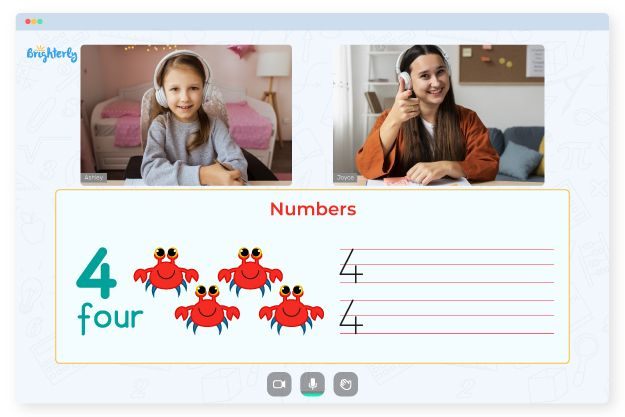# Ordinal Numbers Worksheets

The essential aspect of a child’s early development is honing their mathematical abilities, thus, learning how ordinal numbers function is essential to developing this skill. Ordinal numbers indicate the order in which items appear in a list, such as first, second, third, etc. In a competition, for instance, judges use ordinal numbers to describe the order in which sportsmen cross the finish line.

Our daily lives revolve around ordinal numbers, so children need to know what these terms imply and how to use them. To help simplify the process, here is how an ordinal numbers worksheet can be of help.

## Why Tutors from Brighterly Use Ordinal Numbers Worksheet

Brighterly is an online math platform with tutors that help elementary kids understand math better. Instructors help students understand the sequence of positions using ordinal numbers worksheets. While it seems simple, conveying the information to young children may be a little more complicated.

Math for Kids

Is Your Child Struggling With Math?
1:1 Online Math TutoringTutors understand that kids can quickly grasp concepts like counting and addition compared to positions. So, the tutors use necessary resources like ordinal number worksheets and videos to help students understand math easier.

## How to Use an Ordinal Numbers Worksheet in a Fun Way

Using the ordinal numbers worksheet kindergarten kids need makes your lessons more digestible. With a worksheet, kids can do activities like exercises with days of the week and months of the year. For instance, Sunday is the first day of the week, the second day of the week is Monday, January’s the first month, etc.### Ordinal Numbers Worksheets PDF

Ordinal Number Worksheet### Ordinal Numbers Worksheets PDF

Ordinal Number Worksheets### Ordinal Numbers Worksheets PDF

Ordinal Numbers Worksheet### Ordinal Numbers Worksheets PDF

Ordinal Numbers Worksheet PDF

Worksheets introduce learners to ordinal numbers using printable calendar sheets to display the current month’s position concerning the other months. With this resource, there is no need to make teaching ordinal numbers difficult. Kids can practice ordinal numbers using the worksheets every day. An ordinal numbers worksheet for kindergarten uses routine tasks to make students understand the topic.

### More Numbers Worksheets

Struggling with Numbers?• Is your child finding it challenging to master the fundamentals of numbers?
• An online tutor could be of help.

Does your child struggle to understand numbers lessons? Try studying with an online tutor.